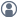###Show Posts

This section allows you to view all posts made by this member. Note that you can only see posts made in areas you currently have access to.

### Topics - k.cerda

Pages: 
1
##### Questions and Answers / Gibbs free energy calculation
« on: October 7, 2021, 13:27 »
Hello

The total energy of a molecule is negative when calculated using TotalEnergy() function: https://docs.quantumatk.com/manual/Types/TotalEnergy/TotalEnergy.html

When I calculate the free (vibrational) energy of the same molecule it is a positive number using the freeEnergy() function: https://docs.quantumatk.com/manual/Types/PhononDensityOfStates/PhononDensityOfStates.html

I want to calculate the Gibbs free energy therefore should I add the total energy obtained from TotalEnergy() and the vibrational free energy obtained from phonon_density_of_states.freeEnergy()? E.g. if the TotalEnergy() gives -20eV and freeEnergy() gives +1eV, is it true that Gibbs free energy of the molecule is -20+1=-19eV?

Thanks a lot.

2
« on: September 26, 2021, 05:47 »
Hello

The total energy gives the total energy  ('Total free energy' at T<> 0 K) and the ground state energy (i.e., at T=0 K). The difference is σS. Is it possible to calculate the total free energy at a broadening other than T=0, e.g. at σ1 using a correction term such as σ1S? Thanks.

3
##### Questions and Answers / possible bug?
« on: August 29, 2021, 07:03 »
I've used counterpoise LCAO calculator to simulate a big structure and took over 36 hrs. I now have the hdf5 file of the converged calculation.

When I use the existing converged hdf5 file to calculate analyses such as electron density, potential and many more, it works as expected, uses the converged data to extract these quantities very fastly.

But when I try to calculate the total energy of the system, ATK doesn't use the converged data to calculate the total energy, instead it re-starts the counterpoise LCAO SCF loops again which takes lots of hours to complete.

Is this a bug or is there a setting to calculate the total energy from converged data of counterpoise corrected LCAO calculation?

Edit: The following total energy calculation triggers a restart of the counterpoise LCAO calculation:

Code
``# -------------------------------------------------------------# Analysis from File# -------------------------------------------------------------path = '/home/kcerda/20082021/converged.hdf5'configuration = nlread(path, object_id='MoleculeConfiguration_0')# -------------------------------------------------------------# Total Energy# -------------------------------------------------------------total_energy = TotalEnergy(configuration)nlsave('/home/kcerda/20082021/convergedTE.hdf5', total_energy)nlprint(total_energy)``

whereas the following calculation uses the converged data and calculates the potential instantly without a restart of SCF loops:

Code
``# -------------------------------------------------------------# Analysis from File# -------------------------------------------------------------path = '/home/kcerda/20082021/converged.hdf5'configuration = nlread(path, object_id='MoleculeConfiguration_0')# -------------------------------------------------------------# Electrostatic Potential# -------------------------------------------------------------electrostatic_potential = ElectrostaticPotential(configuration)nlsave('/home/kcerda/20082021/convergedPOT.hdf5', electrostatic_potential)``

4
##### Questions and Answers / Semi-empirical calculations-basic question
« on: October 10, 2020, 11:09 »
Hi,

I'm just learning to use QuantumATK for my thesis work. I need to simulate organic molecules which contain 5500-15.000 atoms. Therefore DFT does not seem to be feasible due to the large number of atoms. I turned to Semi-Empirical method and it also does not seem to be capable of converging with such number of atoms.  Today I was reading the QuantumATK manual pages and came across the following paragraph:

Quote
"Note

The Hückel parameters have been fitted for non-self-consistent calculations. To use the parameters in self-consistent calculations, the self-consistent onsite shifts must be compensated by a reverse shift of the vacuum_levels."

on page: https://docs.quantumatk.com/manual/SE.html.

Combining this expression with the fact that the no SCF checkbox is checked by default in Semi-Empirical Calculator, can we say that the results of  non-self-consistent calculations with Semi Empirical Calculator in QuantumATK are valid and accurate? In other words, are the results of no SCF Semi-Empirical calculations valid? I understand that self-consistent field is no doubt more accurate but given the very large number of atoms, can the results of non-self-consistent Semi Empirical package be considered valid for a thesis or publication work?

Thanks,
K. Cerda

Pages: